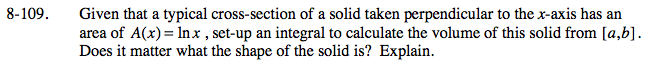### Home > CALC > Chapter 8 > Lesson 8.3.2 > Problem8-109

8-109.

Given that a typical cross-section of a solid taken perpendicular to the x-axis has an area of A(x) = ln x, set-up an integral to calculate the volume of this solid from [a, b]. Does it matter what the shape of the solid is? Explain. Homework Help ✎Of course every shape has a different area function, and this area function = lnx.
It is not possible to know what the cross-sections looks like. Perhaps they are rectangles with height = lnx and base = 1? But they could also be strange looking shapes. Just as long as all of their areas = (coefficient)(base)(height) = lnx, the Volume by Cross Section formula will work.